# RS Aggarwal Solutions for Class 6 Chapter 14 Constructions (Using Ruler and a Pair of Compasses) Exercise 14A

Exercise 14A explains the students about the procedure to construct a perpendicular bisector, angles, line perpendicular to a given line from a point on it and outside it. RS Aggarwal Solutions contains the steps for various constructions which are covered in this chapter. The students can download RS Aggarwal Solutions for Class 6 Chapter 14 Constructions (Using Ruler and a Pair of Compasses) Exercise 14A here.

## Download PDF of RS Aggarwal Solutions for Class 6 Chapter 14 Constructions (Using Ruler and a Pair of Compasses) Exercise 14A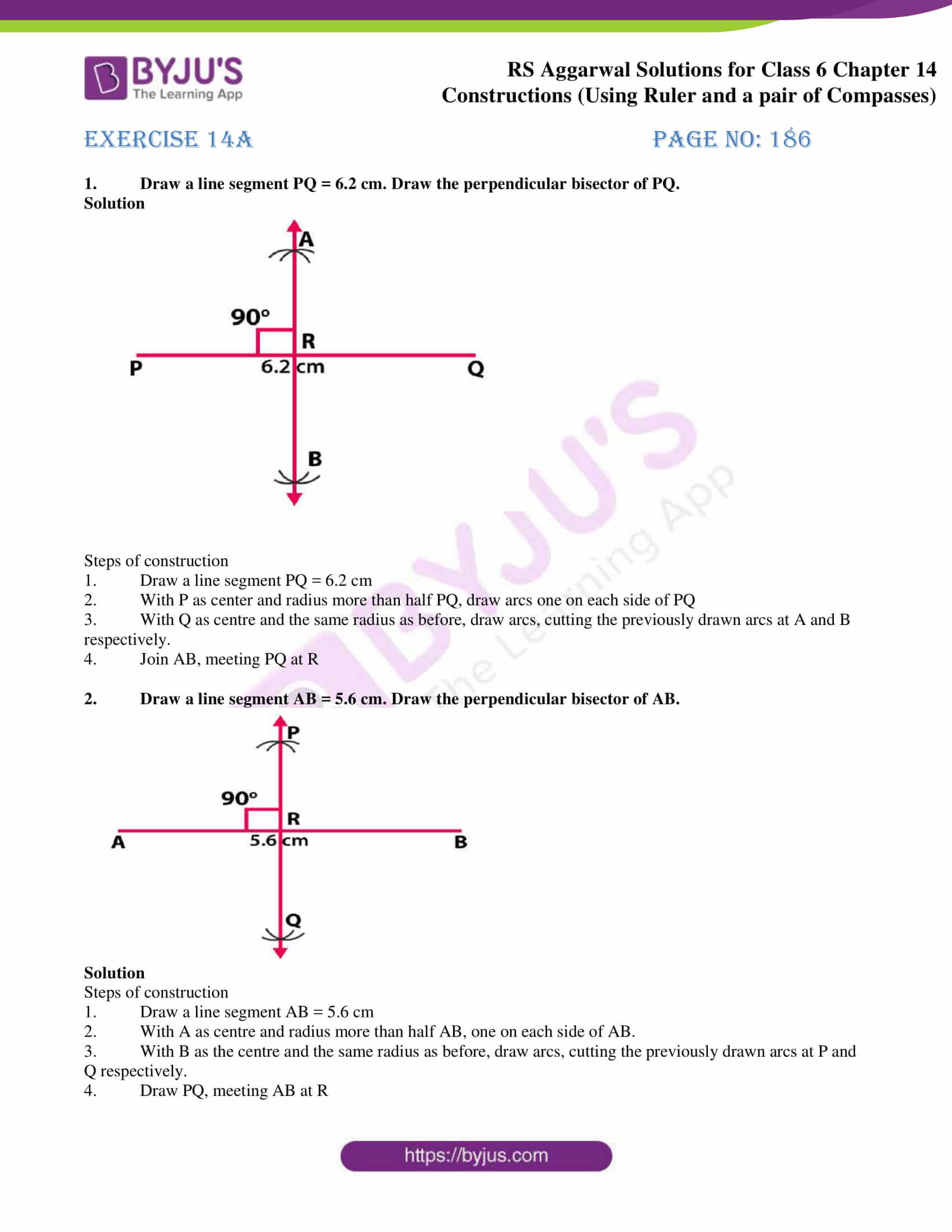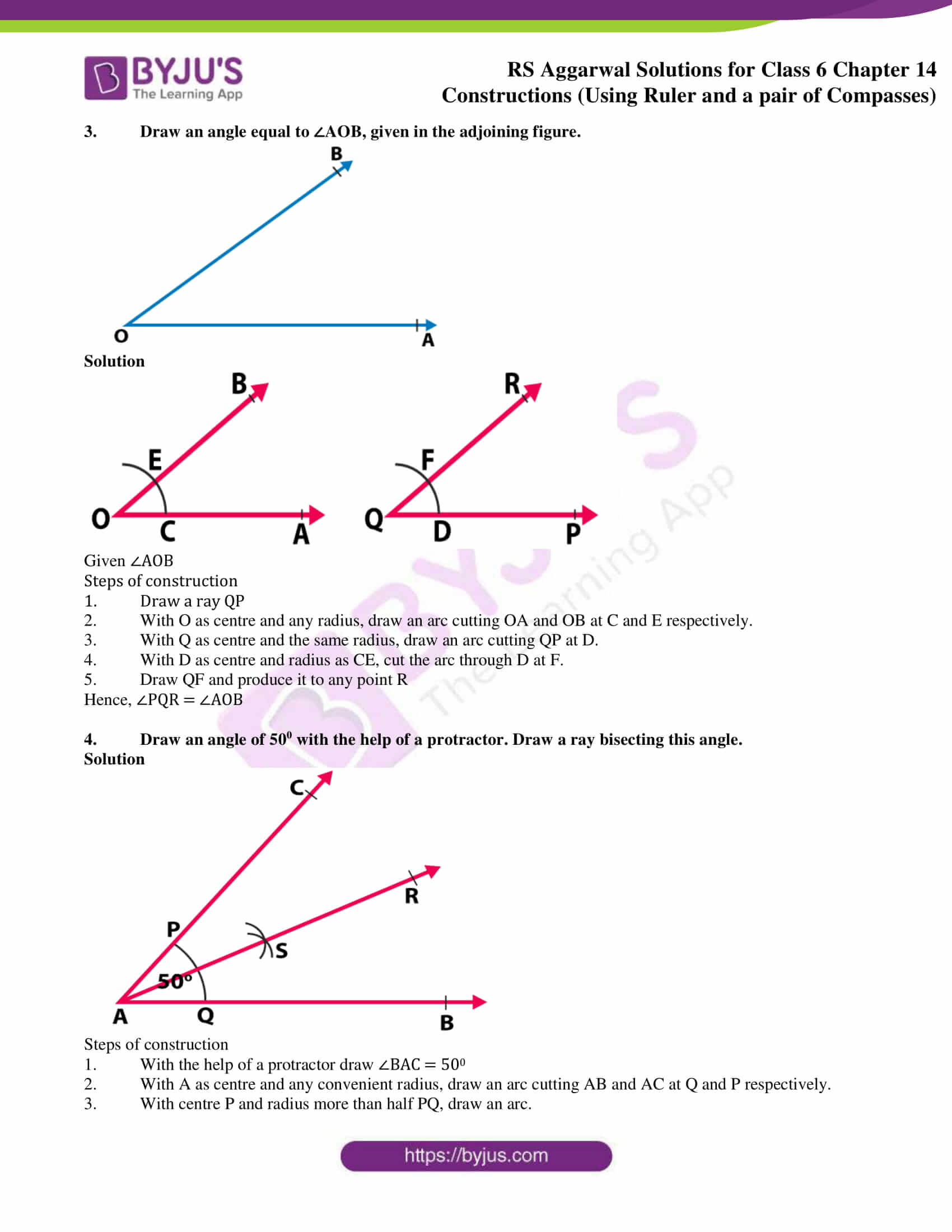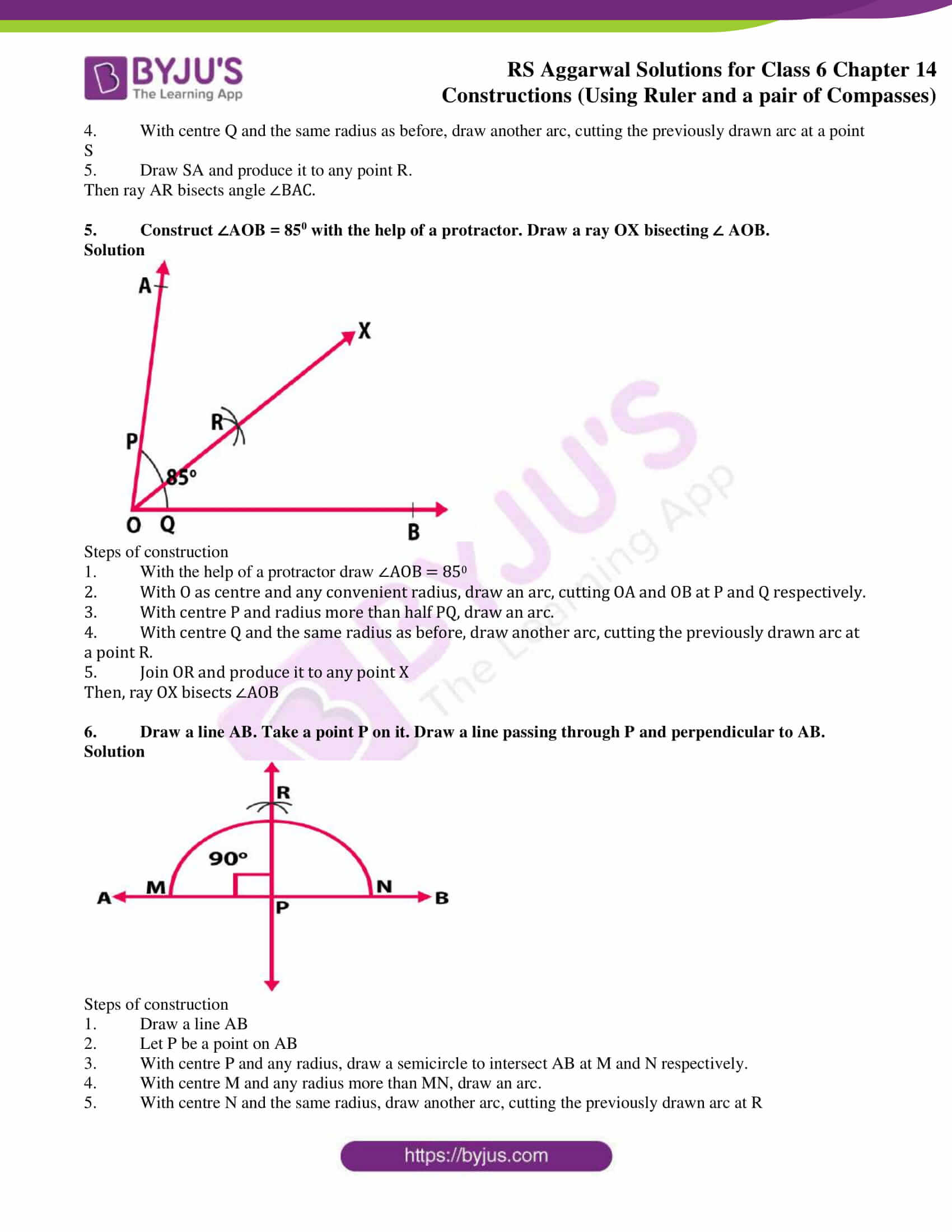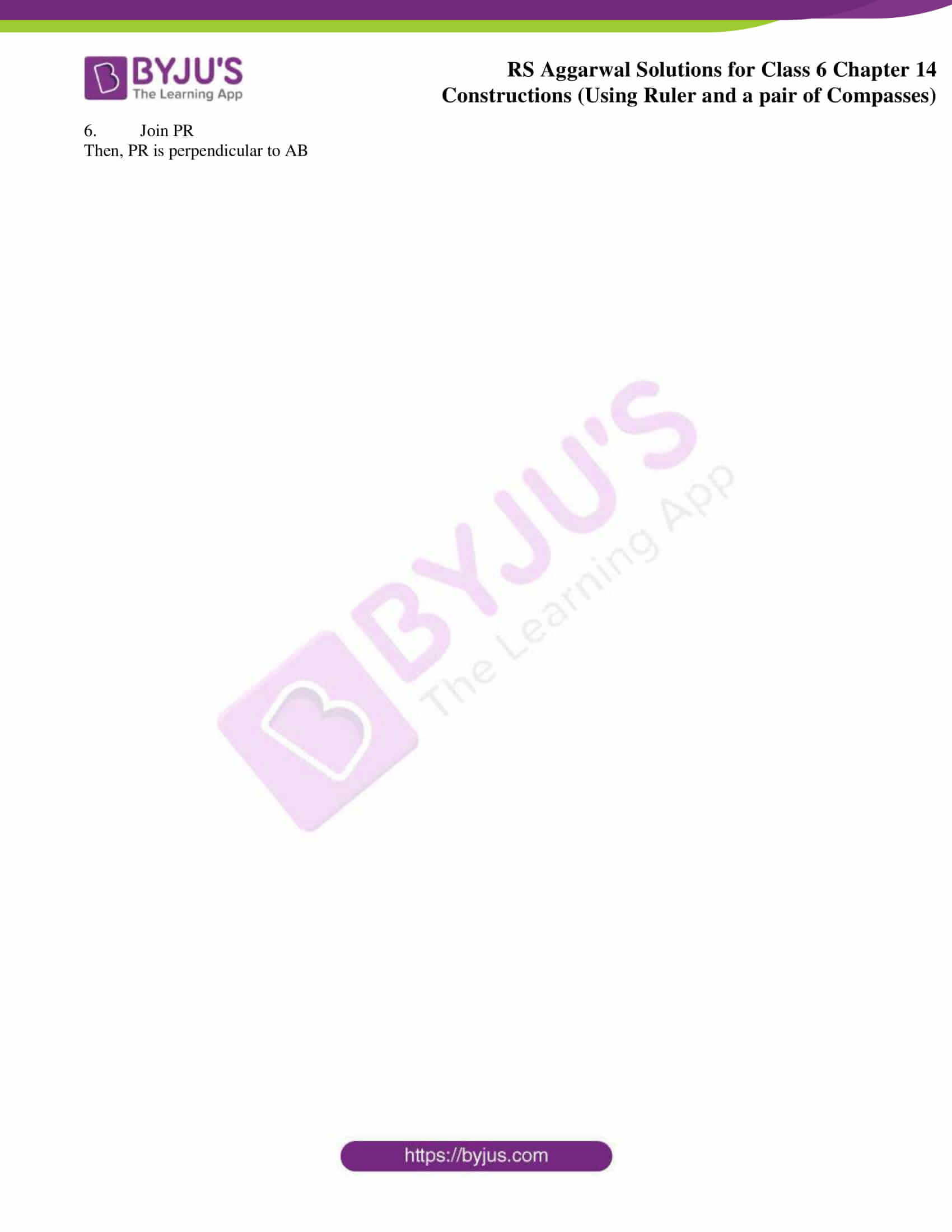### Access answers to Maths RS Aggarwal Solutions for Class 6 Chapter 14 Constructions (Using Ruler and a Pair of Compasses) Exercise 14A

1. Draw a line segment PQ = 6.2 cm. Draw the perpendicular bisector of PQ.

Solution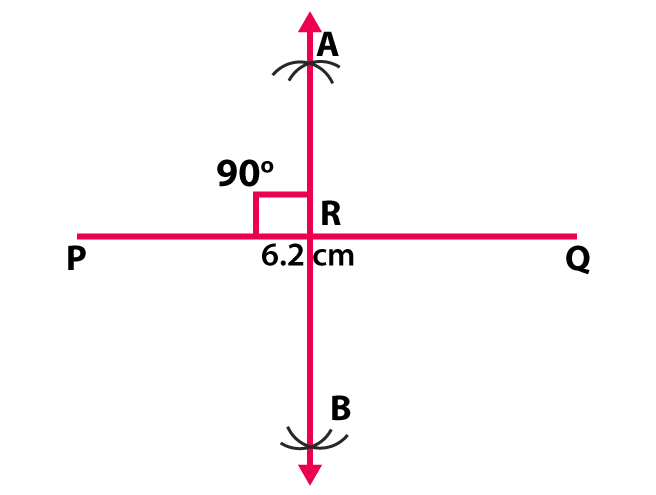Steps of construction

1. Draw a line segment PQ = 6.2 cm

2. With P as center and radius more than half PQ, draw arcs one on each side of PQ

3. With Q as centre and the same radius as before, draw arcs, cutting the previously drawn arcs at A and B respectively.

4. Join AB, meeting PQ at R

2. Draw a line segment AB = 5.6 cm. Draw the perpendicular bisector of AB.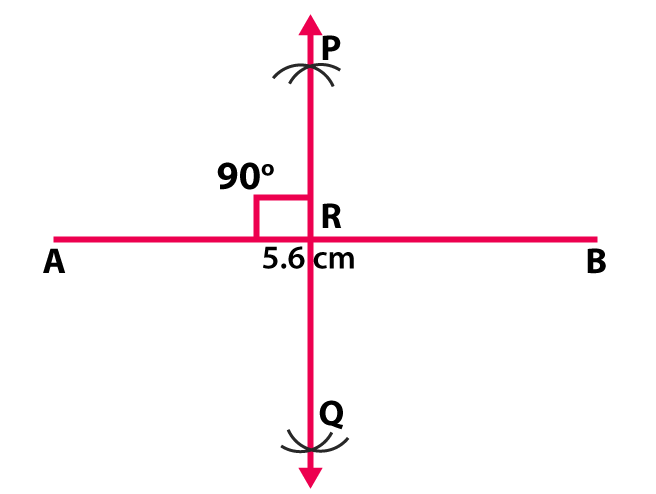Solution

Steps of construction

1. Draw a line segment AB = 5.6 cm

2. With A as centre and radius more than half AB, one on each side of AB.

3. With B as the centre and the same radius as before, draw arcs, cutting the previously drawn arcs at P and Q respectively.

4. Draw PQ, meeting AB at R

3. Draw an angle equal to ∠AOB, given in the adjoining figure.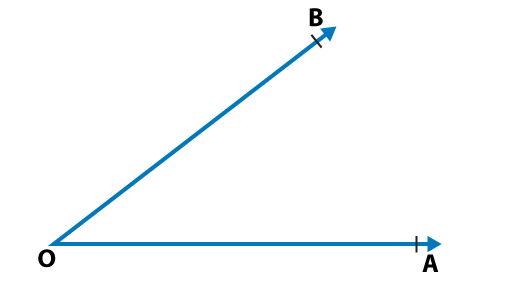Solution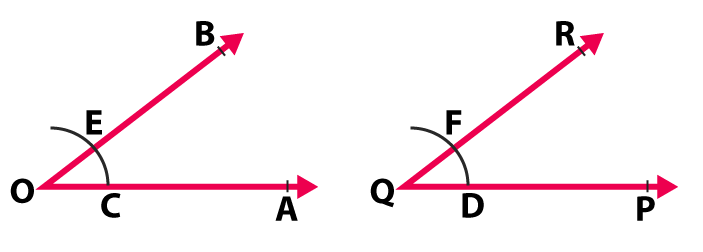Given ∠AOB

Steps of construction

1. Draw a ray QP

2. With O as centre and any radius, draw an arc cutting OA and OB at C and E respectively.

3. With Q as centre and the same radius, draw an arc cutting QP at D.

4. With D as centre and radius as CE, cut the arc through D at F.

5. Draw QF and produce it to any point R

Hence, ∠PQR = ∠AOB

4. Draw an angle of 500 with the help of a protractor. Draw a ray bisecting this angle.

Solution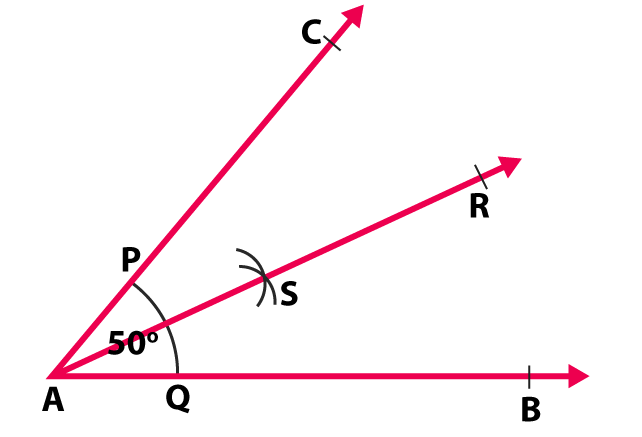Steps of construction

1. With the help of a protractor draw ∠BAC = 500

2. With A as centre and any convenient radius, draw an arc cutting AB and AC at Q and P respectively.

3. With centre P and radius more than half PQ, draw an arc.

4. With centre Q and the same radius as before, draw another arc, cutting the previously drawn arc at a point S

5. Draw SA and produce it to any point R.

Then ray AR bisects angle ∠BAC.

5. Construct ∠AOB = 850 with the help of a protractor. Draw a ray OX bisecting ∠ AOB.

Solution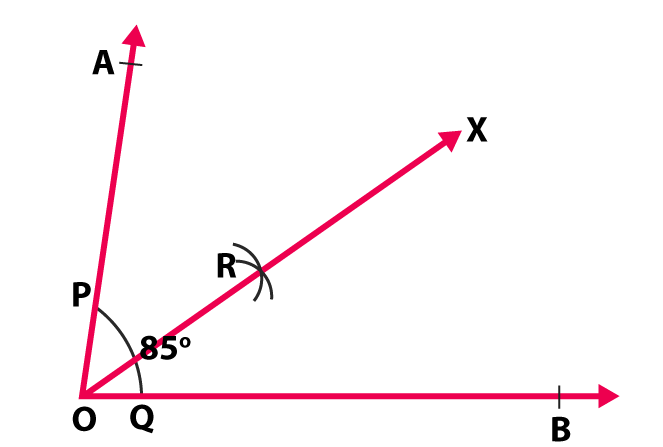Steps of construction

1. With the help of a protractor draw ∠AOB = 850

2. With O as centre and any convenient radius, draw an arc, cutting OA and OB at P and Q respectively.

3. With centre P and radius more than half PQ, draw an arc.

4. With centre Q and the same radius as before, draw another arc, cutting the previously drawn arc at a point R.

5. Join OR and produce it to any point X

Then, ray OX bisects ∠AOB

6. Draw a line AB. Take a point P on it. Draw a line passing through P and perpendicular to AB.

Solution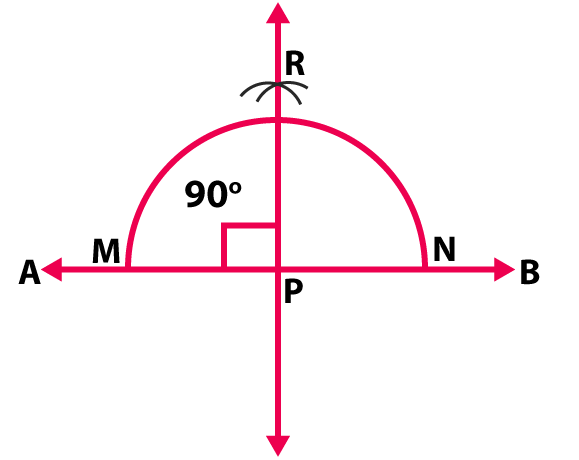Steps of construction

1. Draw a line AB

2. Let P be a point on AB

3. With centre P and any radius, draw a semicircle to intersect AB at M and N respectively.

4. With centre M and any radius more than MN, draw an arc.

5. With centre N and the same radius, draw another arc, cutting the previously drawn arc at R

6. Join PR

Then, PR is perpendicular to AB

### Access other exercises of RS Aggarwal Solutions for Class 6 Chapter 14 Constructions (Using Ruler and a Pair of Compasses)

Exercise 14B Solutions